## 3.2.1 One-Dimensional TAYLOR Expansion

For the sake of brevity, a one-dimensional investigation will be given. The results can be extended straightforwardly to higher dimensions [8, p.150]. Considering a set of grid points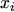the spacing is defined by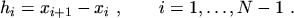(3.1)

Fig. 3.2 shows the notation used.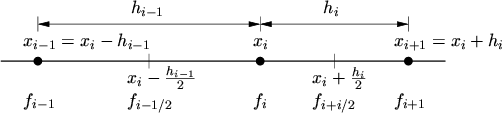For the discretization of the flux equations the derivatives in-between the grid points are important. Therefore a TAYLOR series expansion [47, p.415] around the mid point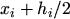is considered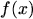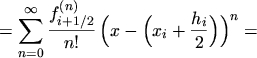(3.2)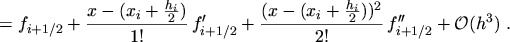(3.3)

To get an expression for the first order derivatives the series up to the order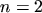is evaluated atand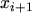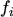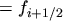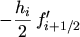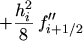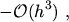(3.4)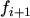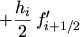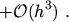(3.5)can be eliminated by subtracting the two equations and thus the first order derivative becomes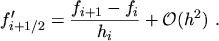(3.6)

For the second order derivatives the TAYLOR series expansion around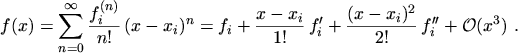(3.7)

is evaluated up to the orderat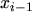and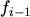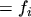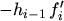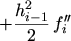(3.8)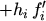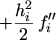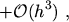(3.9)

and by eliminating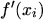the second order derivative is found to be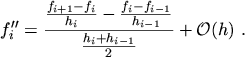(3.10)

No assumption about the uniformity of the grid has been made during the derivation of eqn. (3.6) and eqn. (3.10), so the estimated truncation errors are valid for a non-uniform grid. If a uniform grid spacing is assumed, the truncation error will be of order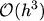in eqn. (3.6) and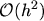in eqn. (3.10) [8, p.153].

M. Gritsch: Numerical Modeling of Silicon-on-Insulator MOSFETs PDF